2017 Month : June Volume : 3 Issue : 2 Page : 27-29

# STRUCTURAL ANALYSIS OF VEDIC MULTIPLIER USING URDHVA-TIRYAKBHYAM SUTRA ALGORITHM FOR FPGA.

Saktiprasanna Swain1, Subhashree Samal2, Manoj Kumar Sahoo3

1M. Tech. Student, Department of Electronics and Communication Engineering, BPUT, Rourkela, Odisha.
2Assistant Professor, Department of Electronics and Communication Engineering, BPUT, Rourkela, Odisha.
3Research Scholar, Department of Electronics and Communication Engineering, BPUT, Rourkela, Odisha.

Corresponding Author:
Manoj Kumar Sahoo,
M-Tech. Student,
Department of Electronics and Communication Engineering,
BPUT, Rourkela, Odisha.
E-mail: mksahoo@hotmail.com, mailymksahoo@gmail.com

ABSTRACT

This paper proposes the design of high speed Vedic multiplier using the techniques of Vedic Mathematics that have been modified to improve performance. A high-speed processor depends greatly on the multiplier as it is one of the key hardware blocks in most digital signal processing systems as well as in general processors. Vedic Mathematics has a unique technique of calculations based on 16 sutras. This paper presents study on high speed 8x8 bit Vedic multiplier architecture, which is quite different from the conventional method of multiplication like add and shift. Further, the Verilog HDL coding of Urdhva-Tiryakbhyam Sutra for 8x8 bits multiplication, squaring circuit as application and their FPGA implementation by Xilinx ISE Tool on Spartan-3E kit have been done and output has been displayed on LEDs of Spartan 3E FPGA board.

KEYWORDS

FPGA, Architecture, Multiplication, Vedic Mathematics, Vedic Multiplier.

Swain S, Samal S, Sahoo MK. Structural analysis of Vedic multiplier using urdhva-tiryakbhyam sutra algorithm for FPGA. J. Technological Advances and Scientific Res.2017;3(2):27-29, DOI: 10.14260/jtasr/2017/08.

Vedic mathematics is the name given to the ancient Indian system of mathematics that was rediscovered in early twentieth century. Vedic mathematics is mainly based on sixteen principles or word-formulae, which are termed as sutras. We discuss a possible application of Vedic mathematics to digital signal processing in the light of application of Vedic multiplication algorithm to digital multipliers. A simple digital multiplier (referred henceforth as Vedic multiplier) architecture based on the Urdhva-Tiryakbhyam (vertically and crosswise) sutra is presented. This sutra was traditionally used in ancient India for the multiplication of two decimal numbers in relatively less time. In this paper, after a gentle introduction of this sutra, it is applied to the binary number system to make it useful in the digital hardware. The hardware architecture of the Vedic multiplier is presented and is shown to be very similar to that of the popular array multiplier. It is also equally likely that many such similar technical applications might come up from the storehouse of knowledge, Veda, if investigated properly. The Vedic mathematical methods suggested by Shankaracharya Sri. Bharati Krishna Tirtha through his book offer efficient alternatives.

Vedic Mathematics (VM)

Vedic mathematics is part of four Vedas (Books of Wisdom). It is part of Sathapatya- Veda (Book on Civil Engineering and Architecture), which is an upaveda (Supplement) of Atharvaveda. It covers explanation of several modern mathematical terms including arithmetic, geometry (plane, coordinate), trigonometry, quadratic equations, factorisation and even calculus. His Holiness Jagadguru Shankaracharya Bharati Krishna Tirthaji Maharaja (1884-1960) comprised all

this work together and gave its mathematical explanation while discussing it for various applications. Swamiji constructed 16 sutras (formulae) and 16 upasutras (subformulae) after extensive research in Atharvaveda. The very word “Veda‟ has the derivational meaning, i.e. the fountainhead and illimitable storehouse of all knowledge. Vedic mathematics is the name given to the ancient system of mathematics or to be precise a unique technique of calculations based on simple rules and principles with which many mathematical problems can be solved, be it arithmetic, algebra, geometry or trigonometry. The system is based on 16 Vedic sutras or aphorisms, which are actually word formulae describing natural ways of solving a whole range of mathematical problems. The beauty of Vedic mathematics lies in the fact that it reduces the otherwise cumbersome-looking calculations in conventional mathematics to a very simple one. This is so because the Vedic formulae are claimed to be based on the natural principles on which the human mind works. This is a very interesting field and presents some effective algorithms, which can be applied to various branches of engineering such as computing and digital signal processing.

Structures of Multipliers

A. Wallace Tree Multiplier

The partial sum adders can also be rearranged in a tree-like fashion reducing both the critical path and the number of adder cells needed. The presented structure is called Wallace tree multiplier and its implementation is in figure. The tree multiplier realises substantial hardware savings for larger multiplier. The propagation delay is reduced as well. In fact, it can be shown that the propagation delay through the tree is equal to O(log3/2(N)). While substantially faster than the carry, save structure for large multiplier words lengths. The Wallace multiplier has the disadvantage of being vary irregular, which complicates the task of an efficient layout design.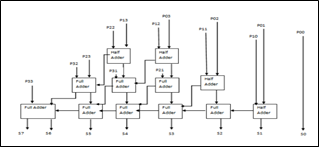Figure 1. Wallace Tree Multiplier Architecture

B. Urdhva-Tiryakbhyam

A Novel Vedic Sutra

The word “Urdhva-Tiryakbhyam” resources vertical and crosswise multiplication. This multiplication formula is pertinent to all cases of algorithm for N bit numbers. Conventionally, this sutra is used for the multiplication of two numbers in decimal number system. The same concept can be applicable to binary number system, which is being discussed in this paper. Advantage of using this type of multiplier is that as the number of bits increases, delay and area increases very slowly as compared to other multipliers.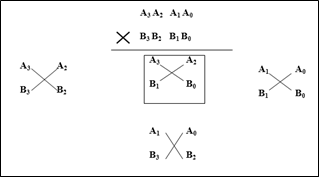Figure 1 (a). Basic Task Segment for

Multiplication Method of Urdhva-Tiryakbhyam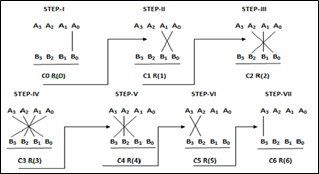Figure.1 (b) Multiplication Method

of Urdhva-Tiryakbhyam

In the above figure-1 (b), 4-bit binary numbers A0A1A2A3 and B0B1B2B3 are considered. The result obtained is stored R0R1R2R3R4R5R6R7.In the first step (A0, B0) is multiplied and the result obtained is stored in R0. Similarly, in second step (A0, B1) and (A1, B0) are multiplied using a full adder and the sum is stored in R1 and carry is transferred to next step. Likewise, the process continues till we get the result.

Proposed Design Structure of Vedic Multipliers

(i) 4-Bit Multipliers

The 4×4 Vedic multiplier in binary is implemented by using VHDL code. In order to reduce the delay of 4×4 multiplier, it is designed by using nine full adders and a 4-bit special adder.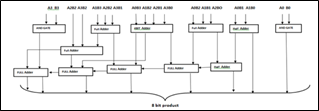Figure 2. 4x4 Multiplication Method of Urdhva-Tiryakbhyam

(ii) 8-Bit Multiplier

The 8×8 Vedic multiplier in binary is implemented using VHDL code. For reducing the delay of 8×8 multiplier, it is implemented using four Vedic 4x4 blocks and a ripple carry adder.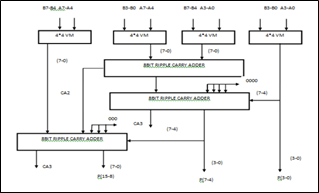Figure 3. 8x8 Multiplication Method of Urdhva-Tiryakbhyam

The 2Nx2N Vedic multiplier in binary is implemented using VHDL code by following structure.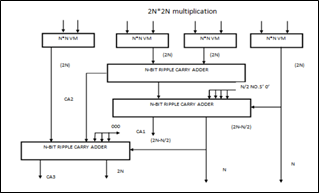Figure 4. 2Nx2N Multiplication Method of Urdhva-Tiryakbhyam

RESULT AND DISCUSSION

The proposed adder and 4x4 Vedic multiplier as well as 8x8 multiplier and squaring circuit is using Urdhva-Tiryakbhyam sutra in binary are implemented using Verilog HDL language and the Vedic computing process is compared with traditional and Wallace tree. The entire code is completely synthesisable. The synthesis is done using Xilinx Synthesis Tool (XST) available with Xilinx ISE13.2. The below figure shows the simulated result with waveforms.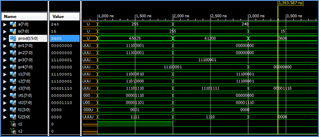Figure 5. Simulation 8x8 Multiplication

Method of Urdhva-Tiryakbhyam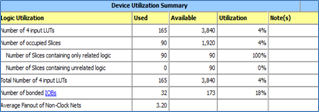Figure 6. Device Utilisation for 8x8 Multiplication

Method of Urdhva-Tiryakbhyam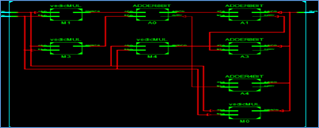Figure 7. 8x8 Multiplication Method

of Urdhva-Tiryakbhyam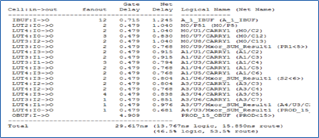Figure 8. Timing Specification for 8x8 Multiplication

Method of Urdhva-Tiryakbhyam

 Design Unit All Delay are in ns (nanosecond) Multiplier Vedic Multiplier Square VM Wallace Tree Multiplier 2x2 6.895 5.895 5.776 6.67 4x4 14.89 12.671 5.934 13.67 8x8 32.617 29.617 14.934 31.7

CONCLUSION

From the result and discussion, it can be concluded that-The Vedic multiplier, Multiplication method of Urdhva-Tiryakbhyam sutra is an effective method for faster multiplication process, which can be used in FPU design unit module. The addition stage can be faster by deploying faster addition process. As the cross steps are reduced to 50%, the squaring circuit delay reduced to almost 50% of Vedic multiplication stages.

REFERENCES

1. Nanda A, Behera S. Design and implementation of Urdhva-Tiryakbhyam based fast 8×8 Vedic binary multiplier.IJERT 2014;3(3).
2. Poornima M, Patil SK, Shivukumar, et al. Implementation of multiplier using Vedic algorithm. JITEE2013;2(6).
3. Premananda BS, Pai SS, Shashank B, et al. Design and implementation of 8-bit Vedic multiplier. JAREEIE 2013;2(12):5877-5882.
4. Anju, AgrawalVK. FPGA implementation of low power and high speed Vedic multiplier using Vedic mathematics.IOSR-JVSP 2013;2(5):51-57.
5. Booth AD. A signed binary multiplication technique. Quarterly Journal of Mechanics and Applied Mathematics 1951;4(2):236-240.
6. Jagadguru Swami Sri Bharathi KrishnaTirathji. Vedic mathematics or sixteen simple mathematical formulae from the vedas.India:MotilalBanarsidas1986.
7. Ramalatha M, Sridharan D. VLSI based high speed karatsuba multiplier for cryptographic applications using Vedic mathematics.IJSCI 2007.
8. CiminieraL, Valenzano A. Low cost serial multipliers for high speed specialised processors. Computers and Digital Techniques. IEEE Proc 1988;135(5):259-265.
9. Verma P, MehtaKK. Implementation of an efficient multiplier based on Vedic mathematics using EDA tool. International Journal of Engineering and Advance Technology (IJEAT) 2012;1(5):2249-8958.
10. Dhillon HS, Mitra A.A reduced- bit multiplication algorithm for digital arithmetics. International Journal of Computational and Mathematical Sciences 2.2 @ www.waset.orgSpring2008.